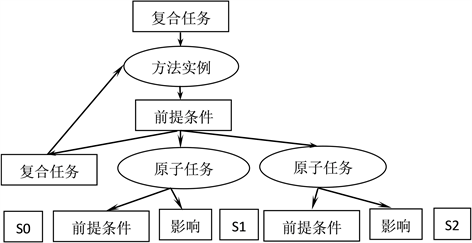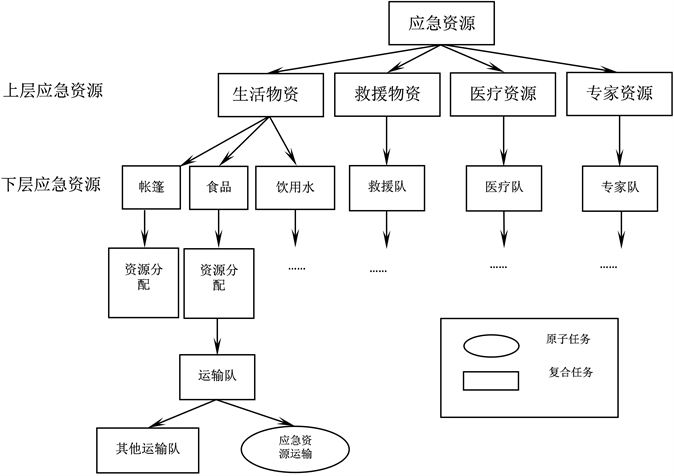#### 期刊菜单

Research on HTN Planning Method for Matching Emergency Logistics Resources and Tasks
DOI: 10.12677/ORF.2022.124166, PDF, HTML, XML, 下载: 37  浏览: 482  科研立项经费支持

Abstract: After the occurrence of an emergency, facing many uncertain factors, using HTN domain knowledge and the principle of planning task decomposition, the emergency action plan was adjusted, identified, and decomposed layer by layer. The compound task was decomposed into atomic actions, and the emergency action plan was finally determined to solve the problem of emergency material allocation and transportation under uncertain conditions. The demand bj, transportation time tij and unit transportation cost cij of the disaster hit points caused by various uncertain factors were expressed as uncertainties. The attributes of the uncertainties were analyzed and a multi-objective model was built. The model was solved by using interval number and triangular fuzzy number theory. The case analysis proved that the emergency time is 138 units shorter than the target time, the total transportation cost was 648 units, and the expected value of safe transportation was 80.2 units, which realized the multi-objective requirements of timeliness, economy and safety.

1. 引言

2. 基本理论

2.1. HTN规划方法

HTN规划任务分解过程：应急行动方案的制定通过HTN规划方法利用领域知识，通过方法实例，递归地将复合任务进行逐层分解，直至分解成为越来越具体原子形式 。图1为分解过程。

2.1.1. 问题描述

${A}_{\text{1}},{A}_{\text{2}},{A}_{\text{3}},\cdots ,{A}_{m}$ 为m个应急物资供应点， ${B}_{\text{1}},{B}_{\text{2}},{B}_{\text{3}},\cdots ,{B}_{n}$ 为n各应急物资需求点，各供应点 ${A}_{i}\left(i=\text{1},\text{2},\cdots ,m\right)$ 的最大供应量为 ${{a}^{\prime }}_{i}$，实际的供应量为ai，各需求点 ${B}_{j}\left(j=1,2,\cdots ,n\right)$ 的需求量为bj (bj为随Figure1. HTN planning task decomposition process

2.1.2. 模型建立

$\mathrm{min}\underset{j=1}{\overset{n}{\sum }}\underset{i=1}{\overset{m}{\sum }}{x}_{ij}\left({\stackrel{˜}{t}}_{ij}-{t}_{j}\right)$ (1)

$\mathrm{min}\underset{j=1}{\overset{n}{\sum }}\underset{i=1}{\overset{m}{\sum }}{\stackrel{˜}{c}}_{ij}{x}_{ij}$ (2)

$\mathrm{max}\underset{j=1}{\overset{n}{\sum }}\underset{i=1}{\overset{m}{\sum }}{P}_{ij}{x}_{ij}$ (3)

$\text{s}\text{.t}\text{.}\text{\hspace{0.17em}}\underset{i=1}{\overset{m}{\sum }}{a}_{i}=\underset{j=1}{\overset{n}{\sum }}{b}_{j}$ (4)

$\underset{j=1}{\overset{n}{\sum }}{x}_{ij}={a}_{i}$ (5)

$\underset{i=1}{\overset{m}{\sum }}{x}_{ij}\ge {b}_{j}$ (6)

$0\le {a}_{i}\le {{a}^{\prime }}_{i}$ (7)

${\stackrel{˜}{t}}_{ij}=\left[{t}_{ij}^{-},{t}_{ij}^{+}\right]$ (8)

${\stackrel{˜}{c}}_{ij}=\left[{c}_{ij1},{c}_{ij2},{c}_{ij3}\right]$ (9)

${b}_{j}~N\left({\mu }_{j},{\sigma }_{j}\right)$ (10)

${x}_{ij}=0$，当 ${p}_{ij}={P}_{0}$(11)

${x}_{ij}\ge 0$ (12)

2.2. 区间数

$\left[a,b\right]+\left[c,d\right]=\left[a+c,b+d\right]$

$\left[a,b\right]-\left[c,d\right]=\left[a-c,b-d\right]$

$k\left[a,b\right]=\left\{\begin{array}{l}\left[ka,kb\right],k\ge 0\\ \left[kb,ka\right],k<0\end{array}$

2.3. 三角模糊数

${I}_{T}\left(\underset{i=1}{\overset{n}{\sum }}\stackrel{˜}{a}\right)=\underset{i=1}{\overset{n}{\sum }}{I}_{T}\left( a ˜ \right)$

${I}_{T}\left(\xi a\right)=\xi {I}_{T}\left(\stackrel{˜}{a}\right)$ ( $\xi$ 为任意实数)

${I}_{T}\left({\stackrel{˜}{a}}_{i}\right)<{I}_{T}\left({\stackrel{˜}{a}}_{j}\right)$，则称 ${\stackrel{˜}{a}}_{i}<{\stackrel{˜}{a}}_{j}$

${I}_{T}\left({\stackrel{˜}{a}}_{i}\right)={I}_{T}\left({\stackrel{˜}{a}}_{j}\right)$，则称 ${\stackrel{˜}{a}}_{i}={\stackrel{˜}{a}}_{j}$

${I}_{T}\left({\stackrel{˜}{a}}_{i}\right)>{I}_{T}\left({\stackrel{˜}{a}}_{j}\right)$，则称 ${\stackrel{˜}{a}}_{i}>{\stackrel{˜}{a}}_{j}$

3. 应急资源分类和特点

3.1. 应急资源分类

1) 人力资源

2) 应急财力资源

3) 应急技术资源

4) 应急设备资源

5) 专用应急物资

3.2. 应急物资特点

1) 不确定性

2) 不可替代性

3) 时效性

4) 滞后性

4. HTN规划分析

4.1. 建立基于层次任务分析HTN的物流管理体系

1) 应急物流管理系统组成

2) 应急物流分类管理的基础

“流体”、“载体”和“流向”是典型的可分类元素。在自然灾害应急物流中，载体通常是中心到地方的应急物资储备、运输枢纽和节点、区域物流中心、社会团体和企业储备等。流动方向通常是救灾现场临时确定的。这两个因素是应急物流的决定因素和稳定因素。对应急物流分类进行管理的概念较为笼统，举例解释应急物流分类进行管理的概念，如：当灾害发生时，需要判断是人为灾害还是自然灾害，一般来讲，自然灾害对应急物流的运用较多，自然灾害发生后，首先根据不同类别判断需求，再根据救援阶段的不同调整应急物资。

3) 应急物流分类管理的依据

4.2. 应急资源任务分解Figure 2. Emergency resource task decomposition tree

5. 案例分析Table 1. Initial task list (original attribute values of roads supplied from supply point Ai to Bj)

$\omega \left(a\right)={a}^{+}-{a}^{-}$

$m\left(a\right)=\left({a}^{-}+{a}^{+}\right)/\text{2}$

${\omega }_{\lambda }\left(a\right)=m\left(a\right)+\lambda \omega \left(a\right)$

${t}_{ij}-{t}_{j}={\omega }_{\lambda }\left(a\right)-{t}_{1}=7-8=-1$Table 2. Road Optimization Attribute Values Supplied from Ai to Bj at Supply Point

$\underset{j=1}{\overset{n}{\sum }}{x}_{ij}={a}_{i}$$0\le {a}_{i}\le {{a}^{\prime }}_{i}$，所以 $\underset{j=1}{\overset{n}{\sum }}{x}_{ij}\le {{a}^{\prime }}_{i}$

${x}_{\text{11}}+{x}_{\text{21}}+{x}_{\text{31}}\ge \text{121}$

$\mathrm{min}T\left(x\right)=-{x}_{11}+{x}_{12}-2{x}_{21}-{x}_{22}+{x}_{23}+{x}_{31}-2{x}_{32}-{x}_{33}$

$\mathrm{min}T\left(x\right)=8.5{x}_{11}+8{x}_{12}+7.5{x}_{13}+7.5{x}_{21}+6.5{x}_{22}+5.5{x}_{32}+6.5{x}_{33}$

$\mathrm{max}S\left(x\right)=0.6{x}_{11}+0.6{x}_{12}+0.7{x}_{13}+0.7{x}_{21}+0.8{x}_{22}+0.9{x}_{32}+0.8{x}_{33}$

$\text{s}\text{.t}.\left\{\begin{array}{l}{x}_{11}+{x}_{12}+{x}_{13}\le 25\\ {x}_{21}+{x}_{22}+{x}_{23}\le 30\\ {x}_{11}+{x}_{21}+{x}_{31}\ge 14.21\\ {x}_{12}+{x}_{22}+{x}_{32}\ge 58.42\\ {x}_{13}+{x}_{23}+{x}_{33}\ge 24.21\\ {x}_{23}={x}_{31}=0\\ {x}_{ij}\ge 0\end{array}$

6. 结论

  王喆, 王世昌, 李明磊, 唐攀. 层次任务网络规划在应急物流方案制定中的应用研究[J]. 安全与环境工程, 2017, 24(5): 15-20.  郝西浩, 张玲. 震后救灾网络中应急资源配置鲁棒优化研究[J]. 武汉理工大学学报(信息与管理工程版), 2019, 41(6): 560-566.  张梦玲, 王晶, 黄钧. 不确定需求下考虑供应商参与机制的应急资源配置鲁棒优化研究[J]. 中国管理科学, 2020, 28(7): 102-111.  王喆, 蒋壮, 王世昌, 刘丹. 应急智能规划中基于约束满足的资源协作方法[J]. 系统工程学报, 2020, 35(6): 816-837.  唐攀, 祁超, 王红卫. 基于层次任务网络规划的应急行动方案制定方法[J]. 管理评论, 2016, 28(8): 43-50.  邵天浩, 张宏军, 程恺, 等. 层次任务网络中的重新规划研究综述[J]. 系统工程与电子技术, 2020, 42(12): 2834-2846.  刘明周, 王强, 凌琳. 基于分层任务网络的云制造任务分解方法[J]. 中国机械工程, 2017, 28(8): 924-930.  王喆, 王世昌, 涂圣友, 等. 应急行动方案决策研究综述[J]. 武汉理工大学学报(信息与管理工程版), 2019, 41(5): 467-472, 484.  张旭, 胡小慧. 面向地震救援的应急物资临时储备库规划研究[J]. 数学的实践与认识, 2020, 50(22): 11-19.  李伟利. 考虑优先级的应急物流系统LRP问题研究[D]: [硕士学位论文]. 北京: 北京交通大学交通运输学院, 2019.